IFT Notes for Level I CFA® Program
IFT Notes for Level I CFA® Program

## 4.1. European Option Pricing

Value of European Option at Expiration

Call option

• At expiration, the value of a call option is the greater of zero or the value of the underlying asset minus the exercise price.
•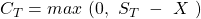Put option

• At expiration, the value of a put option is the greater of zero or the exercise price minus the value of the underlying.
•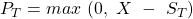Relationship between the value of the option and the value of the underlying

• The value of a European call option is directly related to the value of the underlying.
• The value of a European put option is inversely related to the value of the underlying.

Effect of the Exercise Price

Consider two call options with similar attributes (time to expiration, underlying) but different exercise prices. Assume the exercise price for call option 1 is 25 and that for call option 2 is 30. The right to buy at a lower price of 25 will be more valuable than the right to buy at a higher price of 30.

Similarly, in the case of a put option, the right to sell for a higher price is more valuable than the right to sell for a lower price.

Relationship between the value of the option and the exercise price

• The value of a European call option is inversely related to the exercise price.
• The value of a European put option is directly related to the exercise price.

Moneyness of an option: Indicates whether an option is in, at or out of the money. The table below shows the moneyness of an option for various relative values of S and X.

 Call Option Put Option In-the-money S > X S < X At-the-money S = X S = X Out-of-the-money S < X S > X

Effect of Time to Expiration

Longer-term options should be worth more than shorter-term options. For instance, you have a bought a call option on a stock at an exercise price of $25. Which one would be more valuable to you: an option that expires in a week or an option that expires a year later? Without doubt, the option that expires a year later as it gives enough time for the stock price to increase and make the option valuable. But, is it true for a put option? Partly yes. The more the time to expiration, the more the opportunity for the stock to fall below its exercise price. What does the put option holder get in return when the underlying falls below the exercise price? Just the exercise price. The longer the holder waits to exercise the option, the lower the present value of the payoff. If the discount rate is high, then a longer time to expiration results in a lower present value for the payoff. Relationship between the value of the option and the time to expiration • The value of a European call option is directly related to the time to expiration. • The value of a European put option can be directly or inversely related to the time to expiration. Inversely related under these conditions: the longer the time to expiration, the deeper the option is in the money, and the higher the risk-free rate. Effect of the Risk-free Rate of Interest If the risk-free rate is high, then the call price is higher. For example, you want to invest in a stock whose price is$100. You can either buy the stock for $100 or buy the call option on the stock for$5. Both these actions give you exposure to the stock. If you buy the call option, then you can invest the remaining \$95. If the interest rates are high, then buying the call option is more valuable because of the interest earned.

In the case of puts, the longer the time to expiration with a higher risk-free rate lowers the present value of the exercise price when the option is exercised. That is, when the time to expiration is longer, you get the money later and if the risk-free rate is high, then it is discounted by a higher number which lowers the value of the payoff.

Relationship between the value of the option and the risk-free interest rate

• The value of a European call option is directly related to the risk-free interest rate.
• The value of a European put option is inversely related to the risk-free interest rate.

Effect of Volatility of the Underlying

Volatility is good for both call and put options. If the underlying stock becomes more volatile, then the probability of the options expiring deep in-the-money becomes greater.

Relationship between the value of the option and the volatility of the underlying

• The value of a European call option is directly related to the volatility of the underlying.
• The value of a European put option is directly related to the volatility of the underlying.

Effect of Payments on the Underlying and the Cost of Carry

 Call option Put option Benefits (dividends on stocks, interest on bonds, convenience yield on commodities) Stocks and bonds fall in value when dividends and interest are paid. Decreases because call holders do not get these benefits. Increases Carrying costs Increases as call holders do not incur these costs. Decreases

Relationship between the value of the option and benefits/costs of the underlying

• A European call option is worth less the more benefits that are paid by the underlying. The call option is worth more the more costs that are incurred in holding the underlying.
• A European put option is worth more the more benefits that are paid by the underlying. The put option is worth less the more costs that are incurred in holding the underlying.

Lowest Price of Calls and Puts

In this section, we look at the least price one would be interested in paying for a call option. Let us consider a call option on a stock with a strike price of X that expires at time T. The initial price of the underlying at time t = 0 is , the underlying price at expiration is , and the risk-free rate is r%. If the option is in the money, the payoff at expiration will be ST – X. Lowest price of the call option is given by: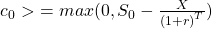Interpreting the above equation:

• It is based on the premise that a call option is equivalent to buying the asset by borrowing the present value of X at a risk-free rate of r%.
• It is the present value of X because you would have to pay X and take delivery of the asset at time T. The payoff for this transaction is ST – X (this number can even be negative). In the case of a call option, it can either be zero (out-of-the-money) or ST-X.
• The price of a call option must be at least zero and not a negative value because if it is less than zero, then it cannot be exercised.
•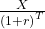is the present value of the exercise price X. So, the lowest price of a call option is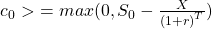Similarly, a put option is equivalent to selling short an asset and investing the proceeds (present value of X) in a risk-free bond at r% for T periods. It will pay X at expiration. A put can never be worth less than zero as the holder cannot be forced to exercise it. The lowest price for a put option is given by: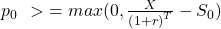Put-Call Parity for European Options

The four instruments in a put-call parity are as follows:

• Asset: An asset such as a stock with no carrying costs and no interim cash flows. At time 0, the price of the stock is S0
• Zero-coupon bond: A zero-coupon bond with a face value of X that matures at time T. At time 0, the value of this bond is.
• Call option: A call option on the stock with a strike price of X, expires at time T. ST is the underlying price at expiration T.
• Put option: A put option on the stock with a strike price of X, expires at time T.

Note: the strike price X of the options is the same as the par value of the bond.

According to put-call parity, fiduciary call = protective put.

A protective put is like buying insurance for an asset you own, specifically a stock, to protect against a downside. A protective put is to buy the underlying and buy a put option on it. For instance, you own 1,000 shares of Apple, the market is highly volatile and you are worried about a huge decline in the near term. To limit the downside risk, you can buy a put option on the stock by paying a premium. This is called a protective put.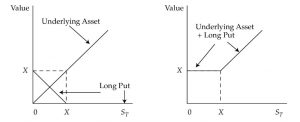Interpretation:

• As you can see, the downside risk is limited to the premium paid for the put. If the underlying price falls below X, it can be still be sold at X by exercising the put option.
• If the underlying rises above X, then it can be sold at the market price and the put option expires worthless.

A fiduciary call is a long call plus a risk-free bond. The diagram below shows the payoff for a fiduciary call: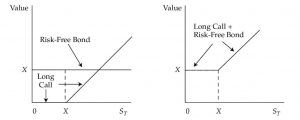The table below shows how the fiduciary call is equal to the protective put under two possible scenarios: when the stock is above the exercise price (call is in-the-money) and the stock is below the exercise price (put is in-the-money).

 Outcome at time T when: à Put expires in-the-money (ST < X) Call expires in-the-money (ST > = X) Protective put Asset ST 0 Long puts X-ST 0 Total X ST Fiduciary call Long call 0 ST – X Risk-free bond X X Total X ST

 Fiduciary Call Protective Put Constituents Long call + risk-free bond Long put + stock Equation c0+p0+S0 Payoff at T if call expires in the money (ST > = X) ST ST Payoff at T if put expires in the money (ST < X) X X Easy way to remember (alphabetical order BCPS) B + C P + S Put-call parity: c0+= p0 + S0

If at time 0, the fiduciary call is not priced the same as the protective put, then there is an arbitrage opportunity. The put-call parity relationship can be rearranged in the following ways:

Synthetic call: c0 = p0 + S0Synthetic bond:= p0 + S0 – c0

Synthetic stock: S0 = c0+– p0

Synthetic put: p0 = c0+– S0

Put-Call Forward Parity for European Options

In this section, we see how to create protective put with a forward contract. Recall we saw in an earlier section that:

Asset – Forward = Risk-free Bond (long asset + short forward = risk-free bond)

Rearranging, we get: Asset = Forward + risk-free bond

Now, if we substitute the asset part in a protective put with the above equation, then we get:

Protective put: Asset + put = Forward + risk-free bond + put

According to put-call parity, since a fiduciary call = protective put, a fiduciary call must be equal to a protective put with a forward contract.

Bond + Call = Long Put + Asset

Replacing S in the above equation with a forward contract, we get:

Bond + Call = Long Put + Long forward + Risk-free bond

The exhibit below shows that the payoffs for fiduciary call is equal to protective put when call and put expire in the money.

 Outcome at T Put Expires In-the-Money (ST < X) Put Expires Out-of-the-Money (ST >= X) Protective put with forward contract Risk-free bond F0(T) F0(T) Forward contract S0 – F0(T) S0 – F0(T) Long put X – S0 0 Total X S0 Fiduciary call Call 0 S0 – X Risk-free bond X X Total X S0